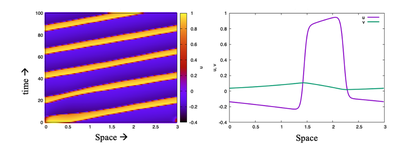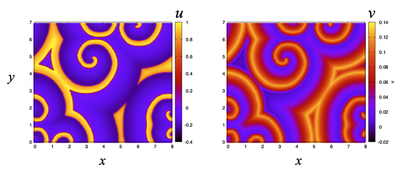# FitzHugh–Nagumo Waves

##### Persistent Identifier

Waves produced by an excitable FitzHugh–Nagumo PDE.

## Introduction

We will here take a closer look at waves produced by an excitable FitzHugh–Nagumo PDE

1. in a periodic 1D domain,
2. in a periodic 2D domain.

## Description

Consider an excitable system such as the FitzHugh-Nagumo model that can produce traveling waves and pulses:

\begin{align} \frac{\partial u}{\partial t} &= D\Delta u + \gamma u(1-u)(u-a) - v + I_0(x,y) \\ \frac{\partial v}{\partial t} &= \epsilon (u-bv) \\ \end{align}

## Results

Simulations of the model in 1D with periodic BoundaryConditions, showing a traveling pulse (top) and in 2D, showing a set of spiral waves (bottom figures).The FitzHugh–Nagumo model in a periodic 1D domain produces a tavelling pulse. Left: kymograph of $u$ over time. Right: the shape of $u$ and $v$ for the pulse. Produced with FNwaves_main.xml.The FitzHugh–Nagumo PDEs can sustain spiral waves when stimulated in a 2D domain. Left: $u$. Right: $v$ at $t = 100$. Produced with FNspiralwaves2D.xml.

## Model

Get this model via:

•  Download: FNwaves_main.xml
• XML Preview

<MorpheusModel version="4">
<Description>
<Title>FNwaves1D</Title>
<Details>Full title:		FitzHugh–Nagumo Waves
Authors:		L. Edelstein-Keshet
Contributors:	Y. Xiao
Date:		23.06.2022
Model ID:		https://identifiers.org/morpheus/M2014
File type:		Main model
Reference:		L. Edelstein-Keshet: Mathematical Models in Cell Biology
Comment:		Waves produced by an excitable FitzHugh–Nagumo PDE in a periodic 1D domain. u is the "voltage", or excitable variable, v is the "refractory" variable. The initial conditions are a peak in u and a shallow ramp in v. The parameter values are as suggested by James Keener.</Details>
</Description>
<Global>
<Constant symbol="L" value="dx*size.x"/>
<Function symbol="x">
<Expression>dx*space.x</Expression>
</Function>
<Field symbol="u" name="u" value="exp(-(x-0.2)^2/0.1)">
<Diffusion rate="0.01"/>
</Field>
<Field symbol="v" name="v" value="0.1*x/L"/>
<DiffEqn symbol-ref="u">
<Expression>H*(-u*(u-1)*(u-a)-v)</Expression>
</DiffEqn>
<DiffEqn symbol-ref="v">
<Expression>eps*(u-b*v)</Expression>
</DiffEqn>
<Constant symbol="eps" value="0.021"/>
<Constant symbol="b" value="2"/>
<Constant symbol="a" value="0.1"/>
<Constant symbol="H" value="20"/>
</System>
</Global>
<Space>
<Lattice class="linear">
<Size symbol="size" value="200, 0, 0"/>
<BoundaryConditions>
<Condition type="periodic" boundary="x"/>
</BoundaryConditions>
<NodeLength symbol="dx" value="0.015"/>
<Neighborhood>
<Order>1</Order>
</Neighborhood>
</Lattice>
<SpaceSymbol symbol="space"/>
</Space>
<Time>
<StartTime value="0"/>
<StopTime value="100"/>
<TimeSymbol symbol="t" name="time"/>
</Time>
<Analysis>
<Logger time-step="1">
<Input>
<Symbol symbol-ref="u"/>
<Symbol symbol-ref="v"/>
<Symbol symbol-ref="L"/>
</Input>
<Output>
<TextOutput/>
</Output>
<Plots>
<Plot time-step="5" title="Spatial profiles">
<Style decorate="true" line-width="3.0" style="lines"/>
<Terminal terminal="png"/>
<X-axis>
<Symbol symbol-ref="x"/>
</X-axis>
<Y-axis>
<Symbol symbol-ref="u"/>
<Symbol symbol-ref="v"/>
</Y-axis>
<Range>
<Time mode="current"/>
</Range>
</Plot>
<Plot time-step="-1" title="Time-space plot">
<Style style="points"/>
<Terminal terminal="png"/>
<X-axis>
<Symbol symbol-ref="x"/>
</X-axis>
<Y-axis>
<Symbol symbol-ref="t"/>
</Y-axis>
<Color-bar>
<Symbol symbol-ref="u"/>
</Color-bar>
</Plot>
</Plots>
</Logger>
<ModelGraph format="dot" reduced="false" include-tags="#untagged"/>
</Analysis>
</MorpheusModel>